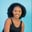Related Tags

python
communitycreator

# How to use Lambda functions to solve problems in PythonMaria Elijah

A lambda function is an anonymous function, which means it is a function without a name.

In Python, a typical function is defined with the def keyword. The lambda keyword is used to define an anonymous function.

### Syntax

lambda arguments: expression


A lambda function can take in any number of arguments, but only one expression.

### Code

#### A lambda function with one argument:

x = lambda y : y + 20
print(x(5))

#### A lambda function with multiple arguments:

Max = lambda y, z : x if(y > z) else z
print(Max(12, 29))

### Problem statement

Find the odd numbers in the list using a lambda function.

### Code

# A python code to find odd numbers in a list
# using Lambda function
# list of numbers from 1 to 11
myList = [1, 2, 3, 4, 5, 6, 7, 8, 9, 10, 11]
# lambda function
odd_nos = list(filter(lambda a: (a % 2 != 0), myList))
print(" Odd numbers in the list : \n", odd_nos) 

### Explanation

In the code above, we create a list of numbers (1 to 11). Next, we use a lambda function to check for odd numbers in the list. Finally, we extract the odd numbers from mylist[] and add them to a new list called odd_nos.

### How to use a Lambda function as an argument to a higher-order function

A higher-order function is a function that passes a function as a parameter to another function, meaning it takes a function (or functions) as an argument. It is a first-class function. The three commonly used higher-order functions are map(), filter(), and reduce().

#### The map() function

map() is used to apply a given function to each element of an iterable and return an iterable of results in the same order. map() takes a function and iterable(s) (e.g. list, tuple) as the second argument that is passed sequentially to the function.

#### Syntax

map(func, iter)

# map() takes Lambda function as an argument
myList = [0, 2, 4, 6, 8, 10]
newList = list(map(lambda x: (x*5), myList))
print("Original list: \n", myList)
print ("The new list: \n", newList)

#### The filter() function

filter() is a function that creates an iterable from an old one by matching every element in the new iterable to a predicate that returns a Boolean value. filter() takes two arguments.

#### Syntax

filter(func, iter)


#### The reduce() function

reduce() is a function that folds or reduces an iterable into a single value. reduce() takes two arguments.

#### Syntax

reduce(func,iter)

# # reduce() takes Lambda function as an argument
from functools import reduce
a = reduce(lambda x, y: x+y, range(1,51))
print("The value of a is ", a)

# using reduce to concatenate string
print(reduce(lambda x, y: x+y, ["Edu", "ca", "tive", " shot"]))

RELATED TAGS

python
communitycreator

CONTRIBUTORMaria Elijah
RELATED COURSES

View all Courses

Keep Exploring

Learn in-demand tech skills in half the time